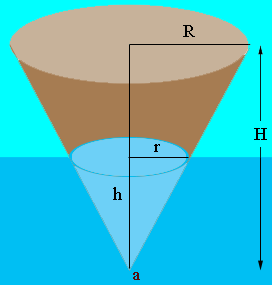SEARCH HOMEMath Central Quandaries & QueriesQuestion from Jean, a student: "A conical buoy that weighs B pounds floats upright in water with its vertex "a" feet below the surface. A crane on a dock lifts the buoy until its vertex just clears the surface. How much work is done?" Thanks, When the crane has lifted the buoy x feet, the force required to hold it in this position is the weight of the buoy minus the upward buoyant force F due to the water still displaced, and I don't know how calculate this force.Hi Jean,

Wikipedia Has a concise statement of Archimedes' principle

Any object, wholly or partially immersed in a fluid, is buoyed up by a force equal to the weight of the fluid displaced by the object.

— Archimedes of Syracuse

The volume of a cone is given by

volume = $\frac13 \pi \times$ radius2 $\times$ height.

Thus from my diagramthe weight of the buoy is

$W_B = \frac13 \pi \times R^2 \times H \times\mbox{ (the density of the buoy material.)}$

The buoyant force is equal to the weight of the fluid displaced by the buoy and this weight is given by

$W_F = \frac13 \pi \times r^2 \times h \times\mbox{ (the density of water.)}$

Thus, at any instance the force required by the crane is $W_B - W_F.$

Does this help? Write back if you need more assistance.

PennyMath Central is supported by the University of Regina and The Pacific Institute for the Mathematical Sciences.